Application of machine learning techniques in estimation of fracture porosity using fuzzy inference system for a FGB reservoir in
Cuu Long basin, Vietnam; Pham Huy Giao, Nakaret Kano, Kushan Sandunil, Bui Duc Trung; Asian Institute of Technology (AIT) Email: hgiao@ait.asia

Summary

Determination of porosity of a fractured granite basement (FGB) reservoir in the Cuu Long basin has always been a challenge for petrophysicists. In this study, an analysis of fracture porosity was successfully conducted, using a machine-learning technique, i.e., fuzzy inference system (FIS), thewell log data including gammaray(GR), deep resistivity (LLD), shallow resistivity (LLS), sonic (DT), bulk density (RHOB), neutron porosity (NPHI), photoelectric factor (PEF) and caliper (CAL) from two wells BHX01 and BHX02, were used as the input for FIS analyses. Fracture porosity calculated by conventional method was found between 0.01 and 2.24% for BHX01 and between 0.15 and 6.63% for BHX02, respectively. These values match very well with those predicted by various FIS techniques, i.e. Sugeno, Mamdani and Subtractive FIS models. It is expected that the approach of using FIS in petrophysical analysis as presented in this paper can be further applied for other fractured granite basement reservoirs in the Cuu Long and Nam Con Son basins, offshore southern Vietnam.

Key words: Machine learning, fuzzy interference system (FIS), well log analysis, fracture porosity, Cuu long basin.

1. Introduction

As shown in Figure 1 the study site is located in the northern Cuu Long basin, which was formed by the fragmentation, rifting, and subsidence of Pre-Tertiary basement and later filled with the-end-of-Eocene to Pliocene-Quaternary continental, shallow marine and coastal plain deposits.

Common methods used to estimate primary porosity of a clastic reservoir are not always suitable to estimate the fracture porosity. As a result, petrophysicists keep trying to find new approaches to estimate fracture porosity. In this study, porosity of a fractured granite basement reservoir will be estimated by using a soft computing technique known as fuzzy inference system (FIS), whose results will be compared with those calculated based on a conventional method .

Concept of soft computing was first put forward by Zadeh , which is known to include the major methods such as fuzzy logic, evolutionary computation, neural computing, and probabilistic reasoning.

Fuzzy interference systems (FIS) are designed using fuzzy set, which is a kind of mathematical set, where each element of the set has a degree of membership. A fuzzy set does not require a crisp and a clearly defined boundary. It can contain elements with a partial degree of membership. A crisp set of reservoir porosity may include{fair, very good} where the degree of membership of an element (denoted as µ) is either 0 or 1; thus, there can only be one kind of reservoir porosity. For example, in Figure2a, if porosity (φf) is greater than 0.5, reservoir porosity is very good (µ = 1.0), but if porosity is lower than 0.5, it is poor (µ = 0). A Fuzzy set, on the other hand, allows each element to have partial membership; thus, one can write a fuzzy set as {poor, fair, good, very good}. As an example, in Figure 2b, if porosity is 0.4, it is classified as fair.Figure 1. Study location.

Fuzzy inference (reasoning) is the actual process of mapping from a given input to an output using fuzzy logic. Figure 3 shows the architecture of a fuzzy inference system.

As seen in Figure 3 the fuzzifier converts the raw input data into a linguistic variable using the membership function stored in the fuzzy knowledge base. The inference engine further converts the fuzzy input to the fuzzy output using if-then type fuzzy rules. The defuzzifier converts the fuzzy output of the inference engine to crisp using membership functions analogous to the ones used by the fuzzifier.

There are two main types of fuzzy interference methods based on clustering of numerical data, i.e. C-means and Subtractive. The former is a supervised algorithm that can only be used when there is a set of inputs and corresponding outputs to those inputs (training set). The final output of fuzzy C-means is not a FIS but rather a list of cluster centres and several membership grades for each data point. One can use the information returned by the fuzzy C-means routine to build a FIS. Two types of FIS can be modelled using C-means clustering, i.e. Mamdani and Sugeno that are similar in many aspects, and namely, the first two parts of the fuzzy inference process, fuzzifying the inputs and applying the fuzzy operator in the inference engine (Figure 3) are exactly the same. The most fundamental difference between Mamdani type FIS and Sugeno type FIS is the way the crisp output is generated from the fuzzy inputs. While Mamdani FIS uses the technique of defuzzification of a fuzzy output, Sugeno FIS uses weighted average to compute the crisp . So basically, Sugeno model bi-passes the defuzzification. As a result, in Mamdani model there is an output membership function whereas in Sugeno model there is no output membership function. Subtractive is an unsupervised algorithm. It can be utilised when there are no corresponding outputs for a set of input data. This method is focusing on finding regions in the feature space with high densities of data points. The data points within a prespecified, fuzzy radius are then subtracted (removed), and the algorithm proceeds its search for a new point with the highest number of neighbours. The iteration continues until all points have been tested. The quality of the solution depends strongly on the choice of initial values .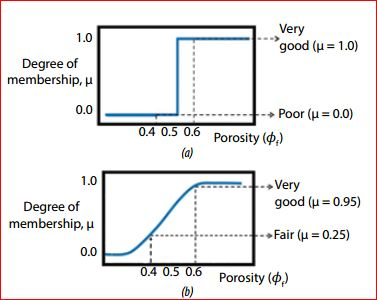Figure 2. Membership function: (a) crisp set; (b) fuzzy set.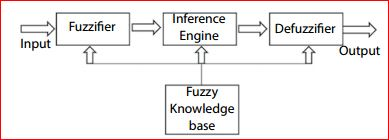Figure 3. Architecture of a fuzzy inference system.

Application of fuzzy interference systems in petrophysics has been used over the past two decades. Fang and Chen  predicted porosity and permeability from the compositional and textural characteristics of sandstones, using fuzzy modelling which is not only assumption-free but also tolerant of outliers. Fuzzy modelling is capable of making both linguistic and numeric predictions based on qualitative knowledge and/or quantitative data. Martinez et al.  presented a technique for the identification and characterisation of naturally fractured reservoirs. A fuzzy inference system was implemented in their study to obtain a fracture index using only data from conventional well logs. Additionally, model from O’Connell and Budiansky  for the prediction of elastic properties of fractured porous rocks is inverted using genetic algorithms to obtain crack density and crack aspect ratio. The results obtained are compared with core information available. Hambalek and Gonzalez  applied fuzzy logic theory in order to establish a narrow relation between well logs and the seven rock types (lithofacies) of the sedimentological model that describes a very complex reservoir in eastern Venezuela. Core analysis of five wells and the established fuzzy relations are used to get the lithofacies description and possible values of permeability in eighteen wells of the same area having only electrical logs. The efficiency of the algorithm developed was verified against a control well with both log and core data. The results are very satisfactory and open the possibilities for future research and application. Abdulraheem  presented the use of fuzzy logic modelling to estimate permeability from wireline logs data for a carbonate reservoir in the Middle East. In this study, correlation coefficients are used as criteria for checking whether a given wireline log is suitable as an input for fuzzy modelling.

2. Methodology

Well log data gamma ray (GR), deep resistivity (LLD), shallow resistivity (LLS), sonic (DT), bulk density (RHOB), neutron porosity (NPHI), photoelectric factor (PEF), and caliper (CAL) were collected from 2 wells BHX01 and BHX02 at a study site in Cuu Long basin, Vietnam.

In this research, the depth interval from 2,515m to 3,015m of well BXH01 and that from 3,050m to 3,870m of well BHX02 were selected. Four reservoir zones of well BHX01 and five reservoir zones of well BHX02  were used in the analyses as shown in Table 1.

The flowchart of the study is shown in Figure 4. First of all, fractureporositieswerecalculatedusingaconventional method suggested by Elkewidy & Tiab  that is also described in detail in Giao and Sandunil  by Equations 1 - 3. Matrix density will be assumed to be 2.71g/cc, which is for limestone, and fluid density is 1.00g/cc, which is for water. This is because parameters of wireline logging tools used to acquire formation density and neutron porosity of formation are calibra and the remaining 25% of the well log data are used for prediction. In the second analysis (Analysis II), the well log data of zones 3 and 4 of BXH01 were used to In the next step, two analyses were conducted to predict porosity using three different FIS models, i.e., Sugeno, Mamdani and Subtractive. In the first analysis (Analysis I), FIS-based prediction was done for every reservoir zone of wells BHX01 and BHX02, separately, i.e., for each zone 75% of well log data are used for training train the FIS models to predict the fracture porosity of zones 1 and 2 of BHX02 as indicated in Table 1.Where:

φt: Total porosity, fraction;

φD: Porosity calculated from bulk density, fraction;

φN: Neutron porosity, fraction; φf: Fracture porosity, fraction; ρb: Bulk density, g/cc;

ρma: Matrix density, g/cc; ρf: Fluid density, g/cc;

m: Cementation factor, dimensionless.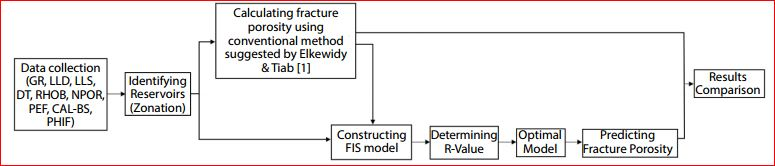Figure 4. Flow chart of the methodology.Table 1. Measured depth and true vertical depth of the analysed reservoir zones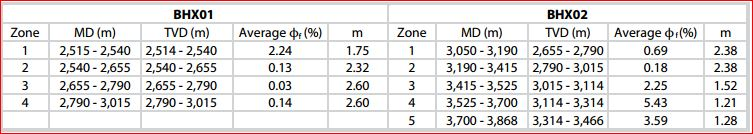Table 2. Fracture porosity calculated by conventional approach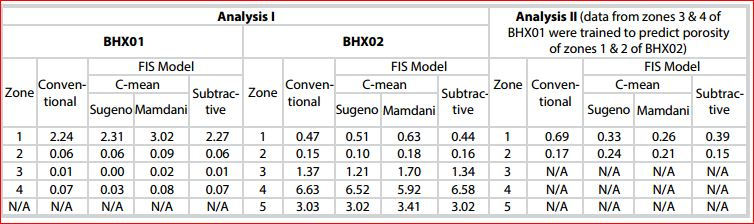Table 3. Results of fracture porosity predicted by FIS in Analyses I and IITable 4. Correlation coefficients in two Analyses I & II, using Sugeno, Mamdani and Subtractive models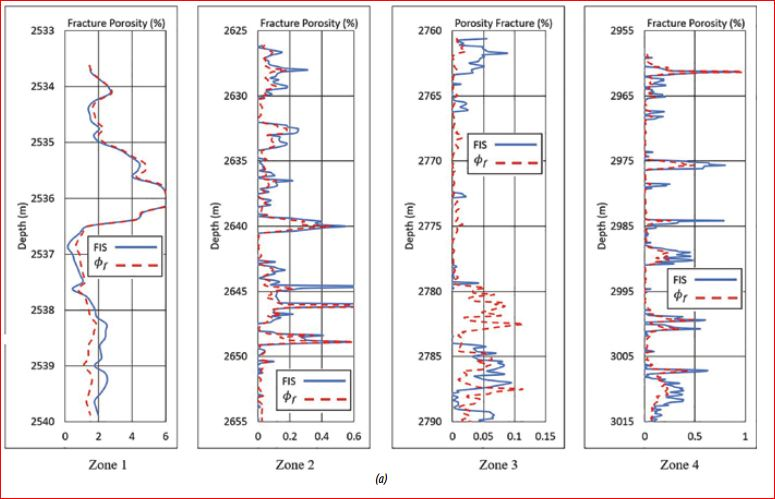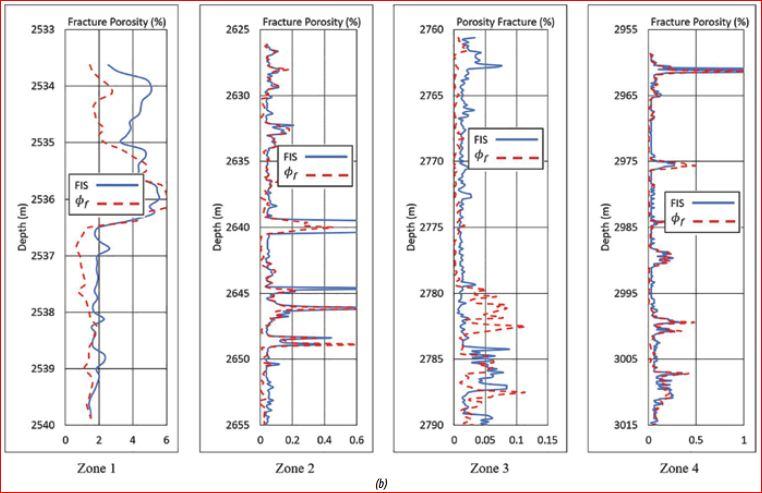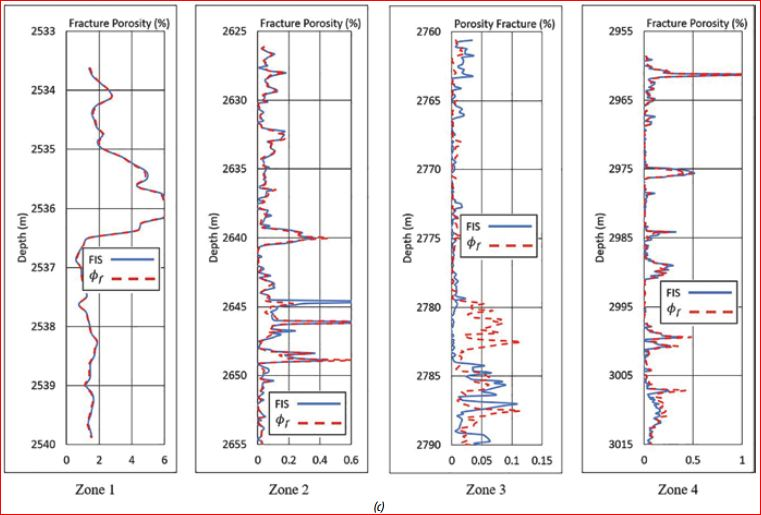Figure 5. Fracture porosity calculated for zones 1 - 4, well BHX01, Analysis I by (a) Sugeno model, (b) Mamdani model, (c) Subtractive model.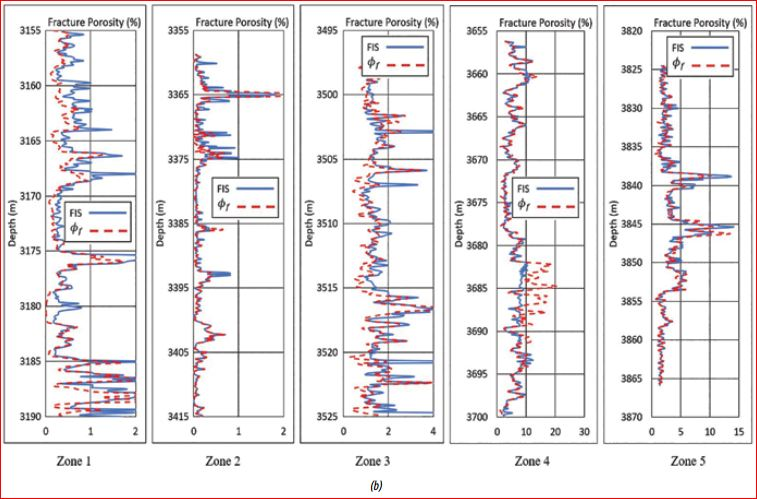Figure 6. Fracture porosity calculated for zones 1 - 5, well BHX02, Analysis I by (a) Sugeno model, (b) Mamdani model, (c) Subtractive model.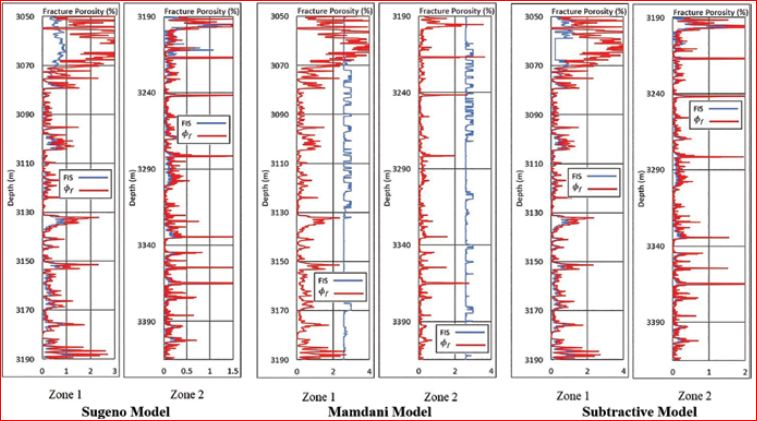Figure 7. Fracture porosity calculated by Sugeno, Mamdani and Subtractive models for zones 1 and 2, well BHX02, Analysis II.

3. Results and discussion

The average fracture porosities for each reservoir zone as calculated using Elkewidy & Tiab’s method  shown in Table 2 are found to be between 0.03 and 2.24 for BHX01, and 0.18 and 5.43 for BHX02, respectively. These conventionally-calculated values were further used to train the FIS models. Table 3 shows the results of Analysis I, indicating the average fracture porosities predicted by Sugeno, Mamdani and Subtractive in comparison with those calculated by the conventional method.

The results of fracture porosity predicted by FIS models are plotted in Figure 5 and Figure 6 as well as shown in Table 3, which are quite close to those calculated by the conventional method shown in Table 2. Among three FIS models, the values predicted by two models of Sugeno and Subtractive are better than those obtained by Mamdani model. This remark is further supported by the calculated correlation coefficient of each FIS analysis as shown in Table 4, which are lower in case of Mamdani model. Out of the three models, Subtractive model gave the best results.

As seen in Figures 5 - 7, the fracture porosity curves predicted by Sugeno and Subtractive models follow well the shape of the conventionally-calculated curve of φf.

4. Conclusions

The FIS Sugeno and Subtractive models proved to be good methods to predict fracture porosity, which is between 0.00 - 2.31 and 0.01 - 2.27 for well BHX01, 0.10 - and 0.16 - 6.58 for well BHX02, respectively.

Fracture porosity predicted by FIS Mamdani are more deviated from the values calculated by Elkewidy & Tiab’s method  comparing to those predicted by  Sugeno and Subtractive models, the fact which is additionally supported by lower values of correlation coefficient.

Out of the three models employed, FIS Subtractive was the best to predict fracture porosity with the highest correlation coefficients.

Although Analysis I that used the well log data in one well to predict fracture porosity prediction for the same well gave better results than Analysis II that used the well data from one well to predict fracture porosity in another well, the results obtained by the latter are also very satisfactory and encouraging for a wider application in practice to predict the porosity of a fractured granite basement reservoirs in the Cuu Long and Nam Con Son basins.

References

1. Tarek Ibrahim Elkewidy, Djebbar Tiab. An application of conventional well logs to characterize naturally fractured reservoirs with their hydraulic (flow) units; A novel approach. SPE 40038. SPE Gas Technology Symposium, Calgary, Canada. 15 - 18 March, 1998.

2. Lotfi A. Zadeh. Fuzzy logic, neural network and soft computing. Communication of the ACM. 1994; 37(3): p. 77- 84.

3. Abdelwahab Hamam, Nicolas D.Georganas. A comparisonof Mamdaniand Sugenofuzzyinferencesystems for evaluating the quality of experience of Hapto-Audio- Visual applications. 2008 IEEE International Workshop on Haptic Audio Visual Environments and Games. 18 - 19 October, 2008: p. 87 - 92.

4. Muriel Bowie. Fuzzy clustering, feature selection, and membership function optimization. Seminar Paper, Department of Informatics, University of Fribourg, Switzerland. 2004.

5. J.H.Fang, H.C.Chen. Fuzzy  modeling  and  the prediction of porosity and permeability from the compositional and textural attributes of sandstone. Journal of Petroleum Geology. 1997; 20(2): p. 185 - 204.

6. L.P.Martinez, R.G.Hughes, M.L.Wiggins. Identification and characterization of naturally fractured reservoir using conventional well logs. University of Oklahoma. 2002.

7. Richard J.O'Connell, Bernard Budiansky. Seismic velocities in dry and saturated cracked solids. Journal of Geophysical Research. 1974; 79(35): p. 5412 - 5426.

8. Nancy Hambalek, Reinaldo Gonzalez. Fuzzy logic applied to lithofacies and permeability forecasting. SPE 81078. SPE Latin America and Caribbean Petroleum Conference, Trinidad. 27 - 30 April, 2003.

9. Abdulazeez Abdulraheem, Emad Sabakhy, Mujahed Ahmed, Aurifullah Vantala, Putu D. Raharja, Gabor Korvin. Estimation of permeability from wireline logs in a Middle East carbonate reservoir using fuzzy logic. SPE 105350. SPE Middle East Oil and Gas Show and Conference, Manama, Bahrain. 11 - 14 March, 2007.

10. Bui Duc Trung. Petrophysical analysis of a fractured granite reservoir, offshore southern Vietnam. Master Thesis, GE-10-12. Asian Institute of Technology (AIT). 2011.

11. Pham Huy Giao, Kushan Sandunil. Application of deep learning in predicting fracture porosity. Petrovietnam Journal. 2017; 10: p. 14 - 22.

Bình luận
 Họ tên Email Mã xác nhận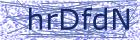Tin khác

Cơ quan chủ quản: Tập đoàn Dầu khí Việt Nam.
Trưởng ban biên tập: Đỗ Chí Thanh, Phó Tổng giám đốc Tập đoàn Dầu khí Việt Nam. .
Số giấy phép: 176/GP-TTĐT, cấp ngày: 11/09/2019. Website: www.pvn.vn
Địa chỉ: Số 18 Láng Hạ, Hà Nội. Tel: (84-4) 38252526. Fax (84-4) 38265942
Phát triển bởi www.paic.pvn.vn